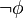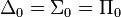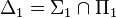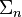# Difference between revisions of "User:IssaRice/Computability and logic/Some important distinctions and equivalences in introductory mathematical logic"

This page lists some important distinctions in introductory mathematical logic and computability theory. Bizarrely, most books won't even mention these distinctions, so you will probably be very confused at the start as you inevitably conflate these ideas.

Completeness The term "complete" can apply to a logic, in which case it is also called "semantically complete". If a logic is semantically complete, it means that if a set of sentences$\Delta$ semantically implies a sentence$\phi$ (i.e. every interpretation that makes every sentence in$\Delta$ true also makes$\phi$ true), then it is possible to prove$\phi$ using$\Delta$ as assumptions. This meaning of completeness is the topic of Gödel's completeness theorem.
The term "complete" can also apply to a theory, in which case it is also called "negation-complete". If a theory is negation-complete, it means that for every sentence$\phi$, the theory proves either$\phi$ or$\neg \phi$ (if it proves both, it is still negation-complete, but it is also inconsistent, so is not an interesting theory). This meaning of completeness is the topic of Gödel's first incompleteness theorem (which states that certain theories of interest are not negation-complete).
Syntax vs semantics I have a rough draft quiz about this that I should post. Also see 
• decides: decidable set/relation vs deciding a sentence vs a theory being decidable vs deciding every sentence vs a logic being decidable (decidability of logic has several equivalent formulations; this is the topic of the Entscheidungsproblem)
• soundness: sound logic (soundness theorem) vs sound theory. soundness of logic is about truth in all interpretations, while soundness of theory is about truth in a specific interpretation. unless the axioms of a theory are just valid sentences (in which case, the axioms are not really adding anything beyond what the logic already has, assuming the logic is complete), the axioms of a theory will in general be false in some interpretations -- this is what makes theories interesting, because they have non-logical content.
• truth in all interpretations (validity) vs truth in the intended interpretation (natural reading, standard interpretation): see User:IssaRice/Computability and logic/Intended interpretation versus all interpretations
• structure vs interpretation vs model: synecdoche problem
• interpretation: there's interpretation in the "structure" sense and also interpretation in the sense of embedding one theory inside another theory (e.g. doing arithmetic with sets)
• theory vs axioms: synecdoche problem
• language vs logic vs formal system vs system
• proves logically vs proves in a particular theory: each theory adds some non-logical axioms that allows it to prove things that aren't logically valid
•$\models$: when a set of sentences comes before the symbol vs when a structure comes before the symbol: see User:IssaRice/Computability and logic/Models symbol
• deciding (corresponding to computable total functions) vs recognizing (semi-deciding, computably enumerating; corresponding to computable partial functions)
• algorithm vs program vs index vs Godel number
• algorithm vs function computed by algorithm: see User:IssaRice/Computability and logic/Function versus algorithm
• expresses vs captures (strong capture, weak capture): see User:IssaRice/Computability and logic/Expresses versus captures
• primitive recursive vs (general, μ) recursive
•$\Delta_0 = \Sigma_0 = \Pi_0$ vs$\Delta_1 = \Sigma_1 \cap \Pi_1$
•$\Sigma_n$ formulas vs relations vs sets
• enumerating vs computably enumerating vs primitively recursively enumerating
• different kinds of deduction systems: axiomatic, natural deduction, sequents, trees
• model as representation vs that which is represented (in mathematical logic, it is that which is represented, but in other disciplines a different meaning of "model" is used)

More speculative:

• different kinds of diagonalization?

There are also some important equivalences to keep in mind:

• Turing computable = computable = recursive = unbounded loops
• Primitive recursive = bounded loops
• computably enumerating = semi-deciding
• computably enumerating in order = deciding
• diagonalization lemma = rogers fixed point theorem
• Kleene's T predicate, godel beta function, Prf(m,n)